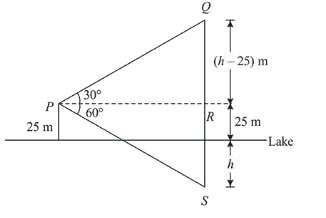# If the angle of elevation of a cloud from aQuestion:

If the angle of elevation of a cloud from a point $\mathrm{P}$ which is $25 \mathrm{~m}$ above a lake be $30^{\circ}$ and the angle of depression of reflection of the cloud in the lake from P be $60^{\circ}$, then the height of the cloud (in meters) from the surface of the lake is:

1. (1) 60

2. (2) 50

3. (3) 45

4. (4) 42

Correct Option: , 2

Solution:

(2) Let height of the cloud from the surface of the lake be $h$ meters.$\therefore \quad$ In $\triangle P R Q:$

$\tan 30^{\circ}=\frac{h-25}{P R}$

$\therefore \quad P R=(h-25) \sqrt{3}$...... (i)

and in $\triangle P R S$ : $\tan 60^{\circ}=\frac{h+25}{P R}$

$P R=\frac{h+25}{\sqrt{3}}$ ..(ii)

Then, from eq. (i) and (ii),

$(h-25) \sqrt{3}=\frac{h+25}{\sqrt{3}}$

$\therefore$ $h=50 \mathrm{~m}$